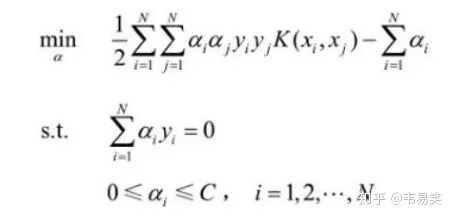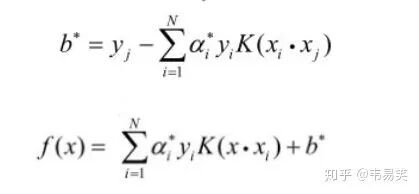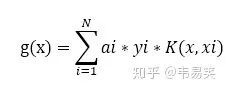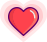### 如何学习SVM？怎么改进实现SVM算法程序？答案来了【导读】在 3D 动作识别领域，需要用到 SVM（支持向量机算法），但是现在所知道的 SVM 算法很多很乱，相关的程序包也很多，有什么方法可以帮助我们更好地理解 SVM 算法，以及如何利用 matlab 编程实现 SVM 算法，同时在理解原有的程序包以及在原有的程序包基础上进行改进有什么好的方法？本文为知乎问题“如何学习 SVM（支持向量机）以及改进实现 SVM 算法程序？”下的两个答案。SVM 的问题就变成：求解一系列满足约束的 alpha 值，使得上面那个函数可以取到最小值。然后记录下这些非零的 alpha 值和对应样本中的 x 值和 y 值，就完成学习了，然后预测的时候用：``target = desired output vector``point = training point matrix``procedure takeStep(i1,i2)``   if (i1 == i2) return 0``   alph1 = Lagrange multiplier for i1``   y1 = target[i1]``   E1 = SVM output on point[i1] – y1 (check in error cache)``   s = y1*y2``   Compute L, H via equations (13) and (14)``   if (L == H)``      return 0``   k11 = kernel(point[i1],point[i1])``   k12 = kernel(point[i1],point[i2])``   k22 = kernel(point[i2],point[i2])``   eta = k11+k22-2*k12``   if (eta > 0)``   {``      a2 = alph2 + y2*(E1-E2)/eta``      if (a2 ``      else if (a2 > H) a2 = H``   }``   else``   {``      Lobj = objective function at a2=L``      Hobj = objective function at a2=H``      if (Lobj ``         a2 = L``      else if (Lobj > Hobj+eps)``         a2 = H``      else``         a2 = alph2``   }``   if (|a2-alph2| ``      return 0``   a1 = alph1+s*(alph2-a2)``   Update threshold to reflect change in Lagrange multipliers``   Update weight vector to reflect change in a1 & a2, if SVM is linear``   Update error cache using new Lagrange multipliers``   Store a1 in the alpha array``   Store a2 in the alpha array``   return 1``endprocedure``

``E(i) = f(xi) - yi``

platt 的文章建议是做一个 E 函数的缓存，方便后面选择 i, j 时比较，我看到很多入门版本 svm 实现都是这么搞得。其实这是有问题的，后面我们会说到。最好的方式是定义一个 g(x) 令其等于：``E(j) = g(xj) + b - yj``

``double Kik = kernel(i, k);``double Kjk = kernel(j, k);``G[k] += delta_alpha_i * Kik * y[i] + delta_alpha_j * Kjk * y[j];``

Platt 的文章里也证明过一旦某个 alpha 出于边界（0 或者 C）的时候，就很不容易变动，而且伪代码里也是优先再工作集里寻找 > 0 and

``K(xi, xj) = xi . xj``

# (*本文为AI科技大本营转载文章，转载请联系作者)推荐阅读

• ## 谁说 C++ 的强制类型转换很难懂？https://www.wxwenku.com/d/201373427